# Selina Solutions Concise Maths Class 10 Chapter 14 Equation of a Line Exercise 14(D)

Finding the slope and y-intercept of a given line is the main topic discussed in this exercise. If students are aspiring to get a good grip in ICSE Class 10 Mathematics, the Selina Solutions for Class 10 Maths is the right tool for this purpose. The solutions are created by our subject experts who have vast academic experience. Selina Solutions Concise Maths Class 10 Chapter 14 Equation of a Line Exercise 14(D) PDF, is provided in the link below.

## Selina Solutions Concise Maths Class 10 Chapter 14 Equation of a Line Exercise 14(D) Download PDF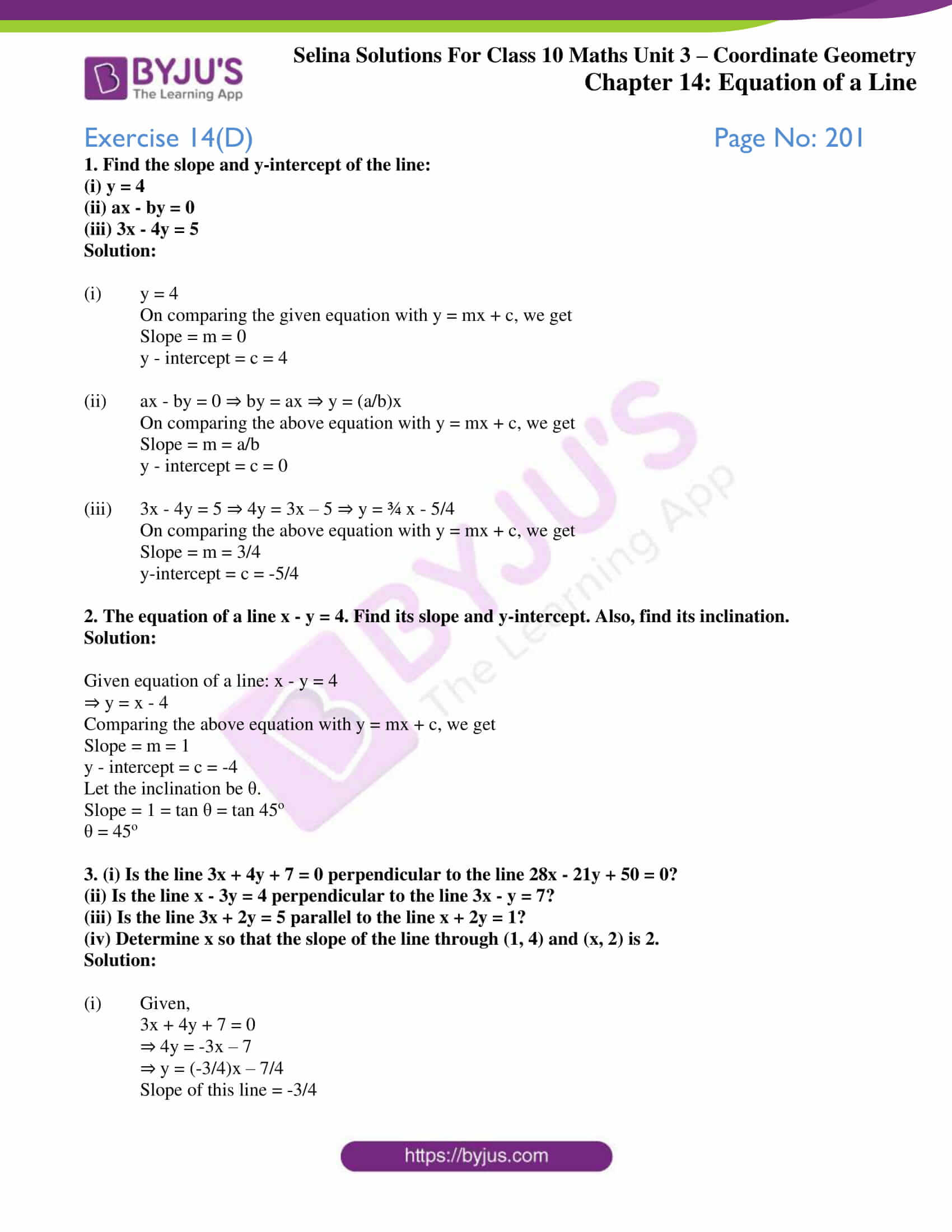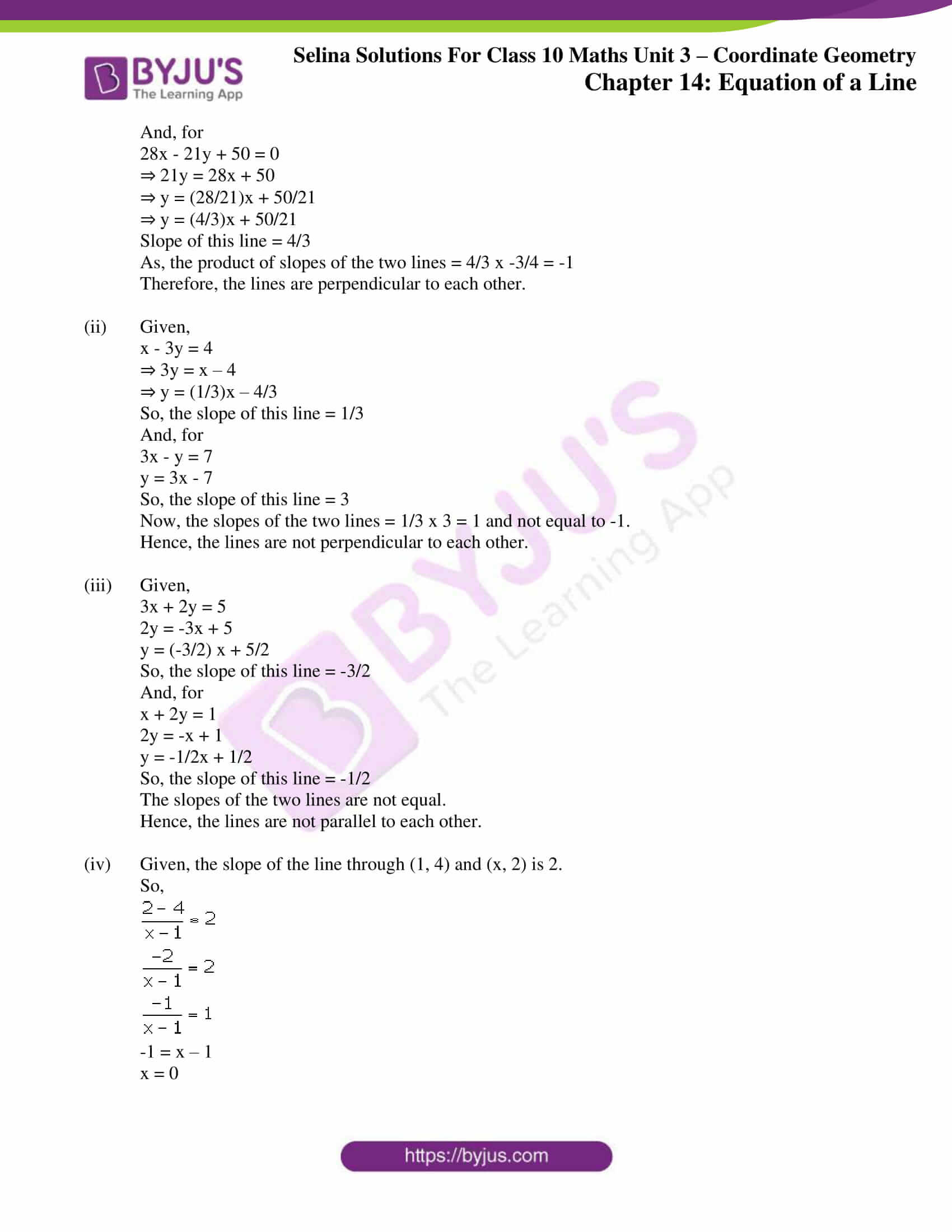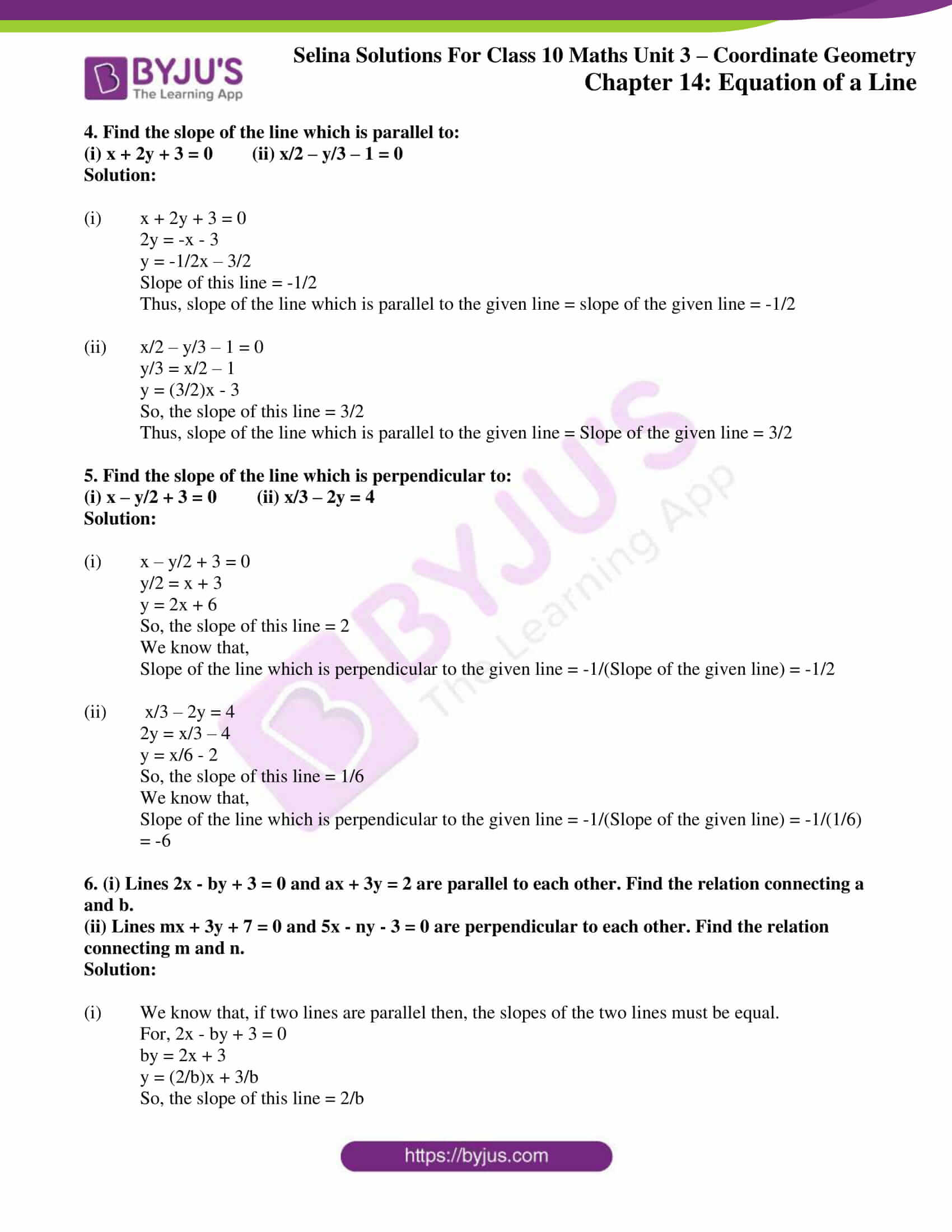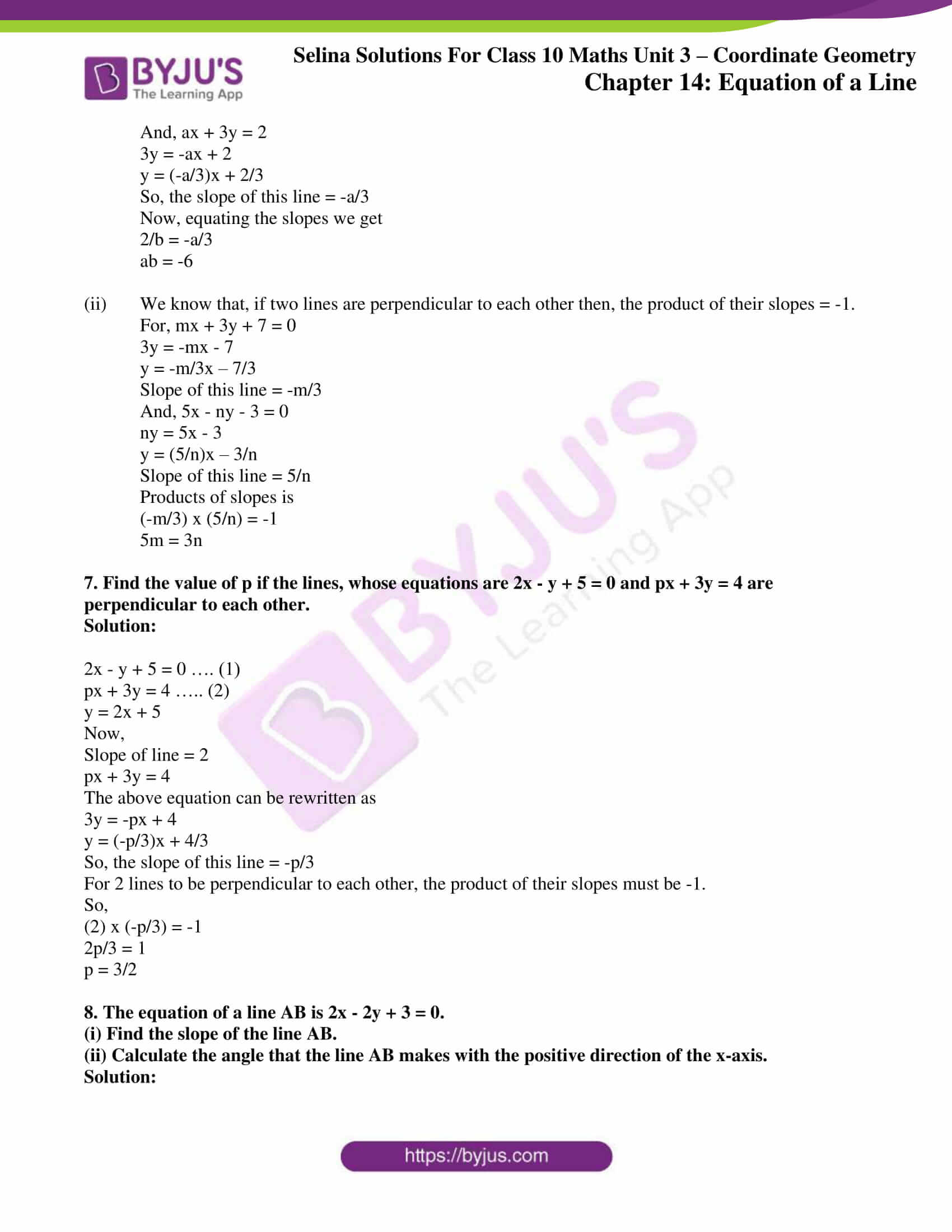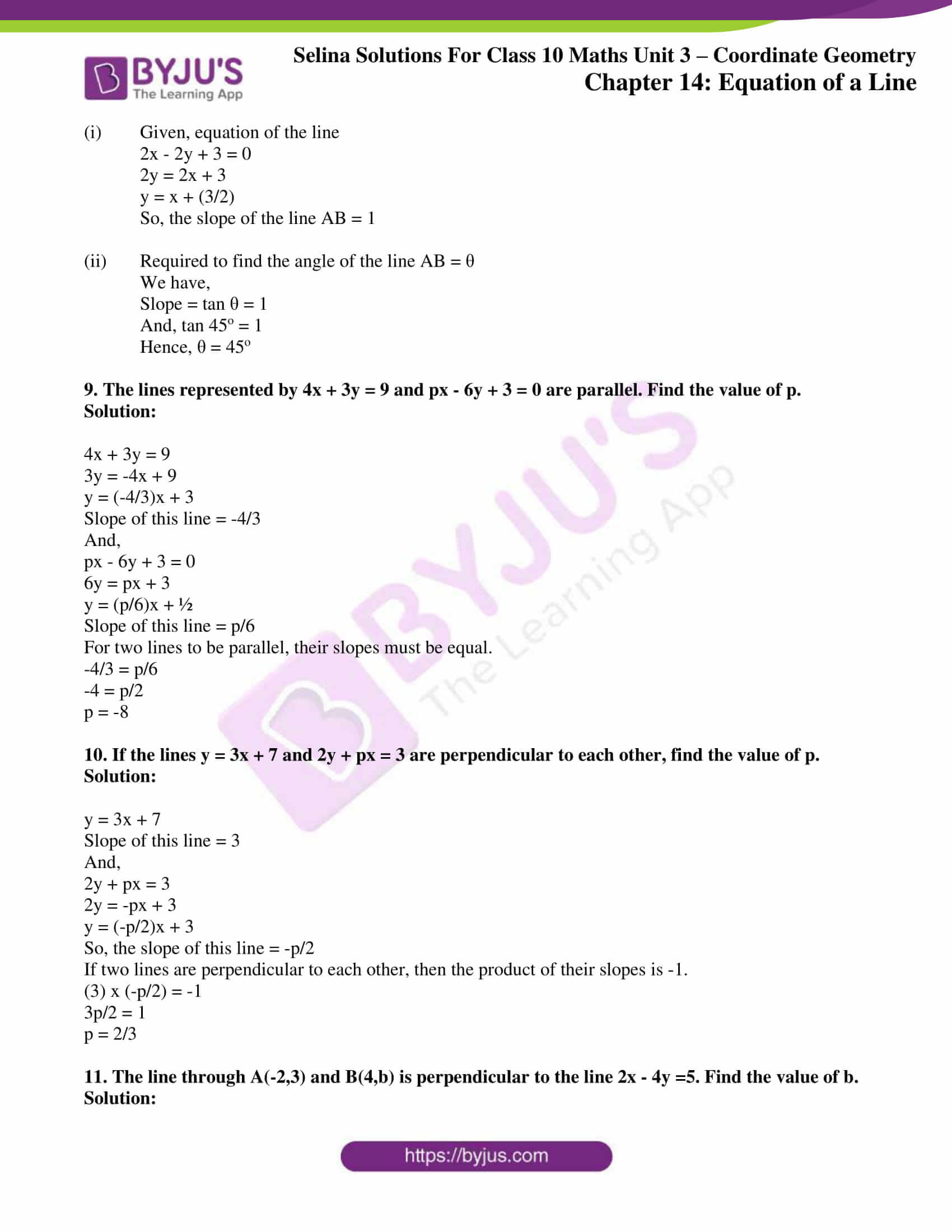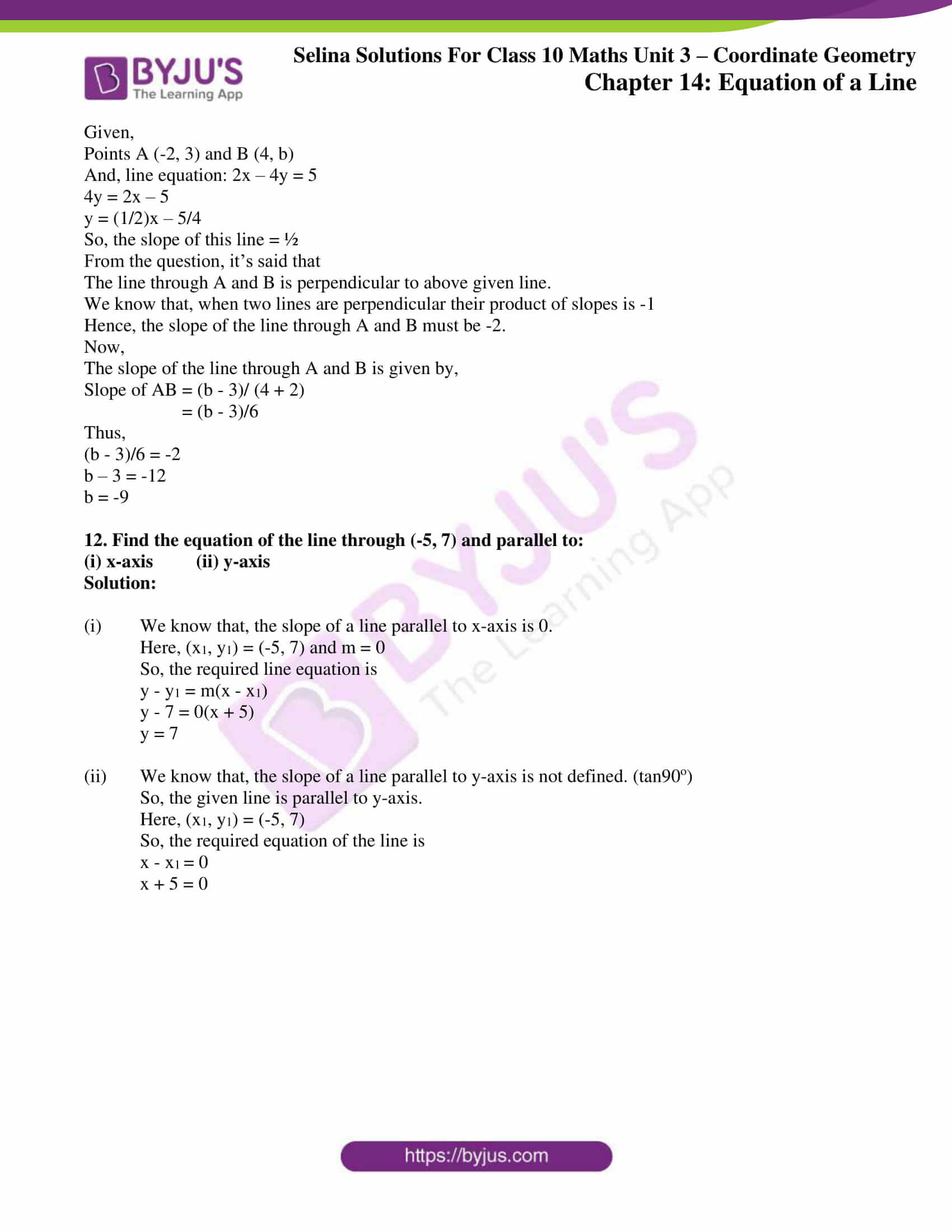### Access other exercises of Selina Solutions Concise Maths Class 10 Chapter 14 Equation of a Line

Exercise 14(A) Solutions

Exercise 14(B) Solutions

Exercise 14(C) Solutions

Exercise 14(E) Solutions

### Access Selina Solutions Concise Maths Class 10 Chapter 14 Equation of a Line Exercise 14(D)

#### Exercise 14(D) Page No: 201

1. Find the slope and y-intercept of the line:

(i) y = 4

(ii) ax – by = 0

(iii) 3x – 4y = 5

Solution:

(i) y = 4

On comparing the given equation with y = mx + c, we get

Slope = m = 0

y – intercept = c = 4

(ii) ax – by = 0 ⇒ by = ax ⇒ y = (a/b)x

On comparing the above equation with y = mx + c, we get

Slope = m = a/b

y – intercept = c = 0

(iii) 3x – 4y = 5 ⇒ 4y = 3x – 5 ⇒ y = ¾ x – 5/4

On comparing the above equation with y = mx + c, we get

Slope = m = 3/4

y-intercept = c = -5/4

2. The equation of a line x – y = 4. Find its slope and y-intercept. Also, find its inclination.

Solution:

Given equation of a line: x – y = 4

⇒ y = x – 4

Comparing the above equation with y = mx + c, we get

Slope = m = 1

y – intercept = c = -4

Let the inclination be θ.

Slope = 1 = tan θ = tan 45o

θ = 45o

3. (i) Is the line 3x + 4y + 7 = 0 perpendicular to the line 28x – 21y + 50 = 0?

(ii) Is the line x – 3y = 4 perpendicular to the line 3x – y = 7?

(iii) Is the line 3x + 2y = 5 parallel to the line x + 2y = 1?

(iv) Determine x so that the slope of the line through (1, 4) and (x, 2) is 2.

Solution:

(i) Given,

3x + 4y + 7 = 0

⇒ 4y = -3x – 7

⇒ y = (-3/4)x – 7/4

Slope of this line = -3/4

And, for

28x – 21y + 50 = 0

⇒ 21y = 28x + 50

⇒ y = (28/21)x + 50/21

⇒ y = (4/3)x + 50/21

Slope of this line = 4/3

As, the product of slopes of the two lines = 4/3 x -3/4 = -1

Therefore, the lines are perpendicular to each other.

(ii) Given,

x – 3y = 4

⇒ 3y = x – 4

⇒ y = (1/3)x – 4/3

So, the slope of this line = 1/3

And, for

3x – y = 7

y = 3x – 7

So, the slope of this line = 3

Now, the slopes of the two lines = 1/3 x 3 = 1 and not equal to -1.

Hence, the lines are not perpendicular to each other.

(iii) Given,

3x + 2y = 5

2y = -3x + 5

y = (-3/2) x + 5/2

So, the slope of this line = -3/2

And, for

x + 2y = 1

2y = -x + 1

y = -1/2x + 1/2

So, the slope of this line = -1/2

The slopes of the two lines are not equal.

Hence, the lines are not parallel to each other.

(iv) Given, the slope of the line through (1, 4) and (x, 2) is 2.

So,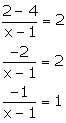-1 = x – 1

x = 0

4. Find the slope of the line which is parallel to:

(i) x + 2y + 3 = 0 (ii) x/2 – y/3 – 1 = 0

Solution:

(i) x + 2y + 3 = 0

2y = -x – 3

y = -1/2x – 3/2

Slope of this line = -1/2

Thus, slope of the line which is parallel to the given line = slope of the given line = -1/2

(ii)  x/2 – y/3 – 1 = 0

y/3 = x/2 – 1

y = (3/2)x – 3

So, the slope of this line = 3/2

Thus, slope of the line which is parallel to the given line = Slope of the given line = 3/2

5. Find the slope of the line which is perpendicular to:

(i) x – y/2 + 3 = 0  (ii) x/3 – 2y = 4

Solution:

(i)  x – y/2 + 3 = 0

y/2 = x + 3

y = 2x + 6

So, the slope of this line = 2

We know that,

Slope of the line which is perpendicular to the given line = -1/(Slope of the given line) = -1/2

(ii)  x/3 – 2y = 4

2y = x/3 – 4

y = x/6 – 2

So, the slope of this line = 1/6

We know that,

Slope of the line which is perpendicular to the given line = -1/(Slope of the given line) = -1/(1/6) = -6

6. (i) Lines 2x – by + 3 = 0 and ax + 3y = 2 are parallel to each other. Find the relation connecting a and b.

(ii) Lines mx + 3y + 7 = 0 and 5x – ny – 3 = 0 are perpendicular to each other. Find the relation connecting m and n.

Solution:

(i) We know that, if two lines are parallel then, the slopes of the two lines must be equal.

For, 2x – by + 3 = 0

by = 2x + 3

y = (2/b)x + 3/b

So, the slope of this line = 2/b

And, ax + 3y = 2

3y = -ax + 2

y = (-a/3)x + 2/3

So, the slope of this line = -a/3

Now, equating the slopes we get

2/b = -a/3

ab = -6

(ii) We know that, if two lines are perpendicular to each other then, the product of their slopes = -1.

For, mx + 3y + 7 = 0

3y = -mx – 7

y = -m/3x – 7/3

Slope of this line = -m/3

And, 5x – ny – 3 = 0

ny = 5x – 3

y = (5/n)x – 3/n

Slope of this line = 5/n

Products of slopes is

(-m/3) x (5/n) = -1

5m = 3n

7. Find the value of p if the lines, whose equations are 2x – y + 5 = 0 and px + 3y = 4 are perpendicular to each other.

Solution:

2x – y + 5 = 0 …. (1)

px + 3y = 4 ….. (2)

y = 2x + 5

Now,

Slope of line = 2

px + 3y = 4

The above equation can be rewritten as

3y = -px + 4

y = (-p/3)x + 4/3

So, the slope of this line = -p/3

For 2 lines to be perpendicular to each other, the product of their slopes must be -1.

So,

(2) x (-p/3) = -1

2p/3 = 1

p = 3/2

8. The equation of a line AB is 2x – 2y + 3 = 0.

(i) Find the slope of the line AB.

(ii) Calculate the angle that the line AB makes with the positive direction of the x-axis.

Solution:

(i) Given, equation of the line

2x – 2y + 3 = 0

2y = 2x + 3

y = x + (3/2)

So, the slope of the line AB = 1

(ii) Required to find the angle of the line AB = θ

We have,

Slope = tan θ = 1

And, tan 45o = 1

Hence, θ = 45o

9. The lines represented by 4x + 3y = 9 and px – 6y + 3 = 0 are parallel. Find the value of p.

Solution:

4x + 3y = 9

3y = -4x + 9

y = (-4/3)x + 3

Slope of this line = -4/3

And,

px – 6y + 3 = 0

6y = px + 3

y = (p/6)x + ½

Slope of this line = p/6

For two lines to be parallel, their slopes must be equal.

-4/3 = p/6

-4 = p/2

p = -8

10. If the lines y = 3x + 7 and 2y + px = 3 are perpendicular to each other, find the value of p.

Solution:

y = 3x + 7

Slope of this line = 3

And,

2y + px = 3

2y = -px + 3

y = (-p/2)x + 3

So, the slope of this line = -p/2

If two lines are perpendicular to each other, then the product of their slopes is -1.

(3) x (-p/2) = -1

3p/2 = 1

p = 2/3

11. The line through A(-2,3) and B(4,b) is perpendicular to the line 2x – 4y =5. Find the value of b.

Solution:

Given,

Points A (-2, 3) and B (4, b)

And, line equation: 2x – 4y = 5

4y = 2x – 5

y = (1/2)x – 5/4

So, the slope of this line = ½

From the question, it’s said that

The line through A and B is perpendicular to above given line.

We know that, when two lines are perpendicular their product of slopes is -1

Hence, the slope of the line through A and B must be -2.

Now,

The slope of the line through A and B is given by,

Slope of AB = (b – 3)/ (4 + 2)

= (b – 3)/6

Thus,

(b – 3)/6 = -2

b – 3 = -12

b = -9

12. Find the equation of the line through (-5, 7) and parallel to:

(i) x-axis (ii) y-axis

Solution:

(i) We know that, the slope of a line parallel to x-axis is 0.

Here, (x1, y1) = (-5, 7) and m = 0

So, the required line equation is

y – y1 = m(x – x1)

y – 7 = 0(x + 5)

y = 7

(ii) We know that, the slope of a line parallel to y-axis is not defined. (tan90o)

So, the given line is parallel to y-axis.

Here, (x1, y1) = (-5, 7)

So, the required equation of the line is

x – x= 0

x + 5 = 0